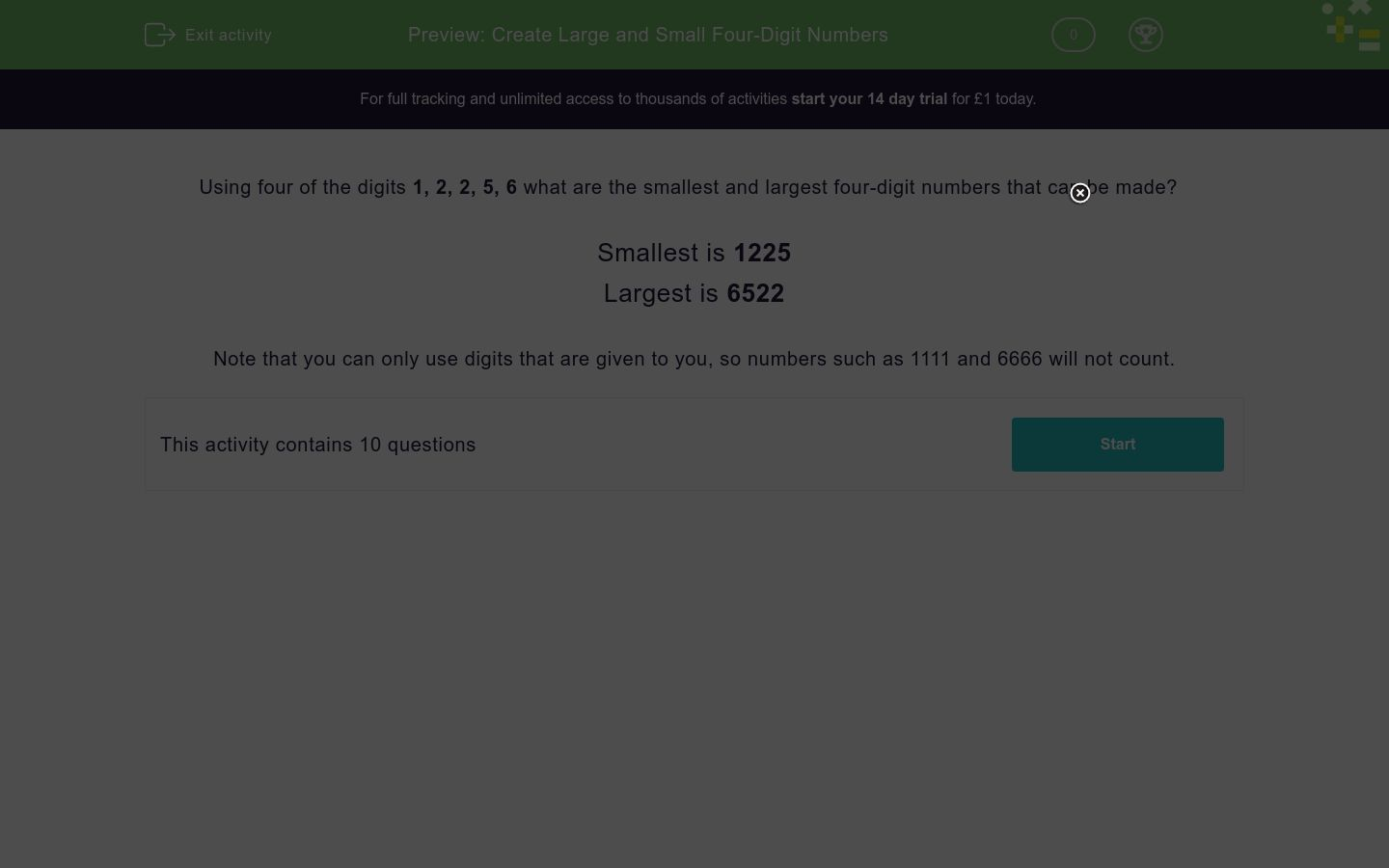# Create Large and Small Four-Digit Numbers

In this worksheet, students are given five digits from which to create the largest and smallest possible four-digit numbers.Key stage:  KS 2

Curriculum topic:   Number: Number and Place Value

Curriculum subtopic:   Order/Compare Numbers Beyond 1000

Difficulty level:### QUESTION 1 of 10

Using four of the digits 1, 2, 2, 5, 6 what are the smallest and largest four-digit numbers that can be made?

Smallest is 1225

Largest is 6522

Note that you can only use digits that are given to you, so numbers such as 1111 and 6666 will not count.

Using four of the digits 1, 3, 2, 5, 3 what are the smallest and largest four-digit numbers that can be made?

 5231 5332 1322 1233 smallest largest

Using four of the digits 1, 4, 5, 4, 5 what are the smallest and largest four-digit numbers that can be made?

 5541 5544 1454 1445 smallest largest

Using four of the digits 1, 2, 7, 3, 4 what are the smallest and largest four-digit numbers that can be made?

 7423 2347 1234 7432 smallest largest

Using four of the digits 1, 8, 3, 5, 9 what are the smallest and largest four-digit numbers that can be made?

 1389 1358 9851 9853 smallest largest

Using four of the digits 4, 9, 4, 2, 9 what are the smallest and largest four-digit numbers that can be made?

 2944 2449 9492 9944 smallest largest

Using four of the digits 4, 6, 4, 4, 4 what are the smallest and largest four-digit numbers that can be made?

 4444 4446 6444 6644 smallest largest

Using four of the digits 4, 6, 4, 6, 6 what are the smallest and largest four-digit numbers that can be made?

 4446 4466 6644 6664 smallest largest

Using four of the digits 8, 6, 4, 4, 6 what are the smallest and largest four-digit numbers that can be made?

 8646 8664 4466 4646 smallest largest

Using four of the digits 8, 6, 9, 1, 1 what are the smallest and largest four-digit numbers that can be made?

 8611 9861 1681 1168 smallest largest

Using four of the digits 8, 6, 9, 1, 9 what are the smallest and largest four-digit numbers that can be made?

 1669 8699 1689 9986 smallest largest
• Question 1

Using four of the digits 1, 3, 2, 5, 3 what are the smallest and largest four-digit numbers that can be made?

 5231 5332 1322 1233 smallest largest
EDDIE SAYS
The smallest number you can make is 1233 and the largest is 5332.
Remember, you can't make 1322 from 1, 3, 2, 5, 3.
• Question 2

Using four of the digits 1, 4, 5, 4, 5 what are the smallest and largest four-digit numbers that can be made?

 5541 5544 1454 1445 smallest largest
EDDIE SAYS
The smallest number you can make is 1445 and the largest is 5544.
• Question 3

Using four of the digits 1, 2, 7, 3, 4 what are the smallest and largest four-digit numbers that can be made?

 7423 2347 1234 7432 smallest largest
EDDIE SAYS
The smallest number you can make is 1234 and the largest is 7432.
• Question 4

Using four of the digits 1, 8, 3, 5, 9 what are the smallest and largest four-digit numbers that can be made?

 1389 1358 9851 9853 smallest largest
EDDIE SAYS
The smallest number you can make is 1358 and the largest is 9853.
• Question 5

Using four of the digits 4, 9, 4, 2, 9 what are the smallest and largest four-digit numbers that can be made?

 2944 2449 9492 9944 smallest largest
EDDIE SAYS
The smallest number you can make is 2449 and the largest is 9944.
• Question 6

Using four of the digits 4, 6, 4, 4, 4 what are the smallest and largest four-digit numbers that can be made?

 4444 4446 6444 6644 smallest largest
EDDIE SAYS
The smallest number you can make is 4444 and the largest is 6444.
Remember, you can't make 6644 out of 4, 6, 4, 4, 4.
• Question 7

Using four of the digits 4, 6, 4, 6, 6 what are the smallest and largest four-digit numbers that can be made?

 4446 4466 6644 6664 smallest largest
EDDIE SAYS
The smallest number you can make is 4466 and the largest is 6664.
Remember, you can't make 4446 from 4, 6, 4, 6, 6.
• Question 8

Using four of the digits 8, 6, 4, 4, 6 what are the smallest and largest four-digit numbers that can be made?

 8646 8664 4466 4646 smallest largest
EDDIE SAYS
The smallest number you can make is 4466 and the largest is 8664.
• Question 9

Using four of the digits 8, 6, 9, 1, 1 what are the smallest and largest four-digit numbers that can be made?

 8611 9861 1681 1168 smallest largest
EDDIE SAYS
The smallest number you can make is 1168 and the largest is 9861.
1681 is larger than 1168.
• Question 10

Using four of the digits 8, 6, 9, 1, 9 what are the smallest and largest four-digit numbers that can be made?

 1669 8699 1689 9986 smallest largest
EDDIE SAYS
The smallest number you can make is 1689 and the largest is 9986. Remember, you can't make 1669 from 8, 6, 9, 1, 9.
---- OR ----

Sign up for a £1 trial so you can track and measure your child's progress on this activity.

### What is EdPlace?

We're your National Curriculum aligned online education content provider helping each child succeed in English, maths and science from year 1 to GCSE. With an EdPlace account you’ll be able to track and measure progress, helping each child achieve their best. We build confidence and attainment by personalising each child’s learning at a level that suits them.

Get started